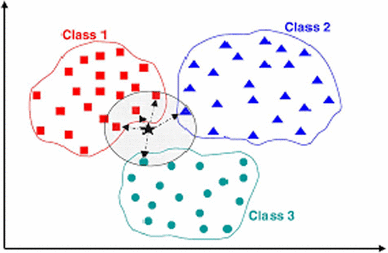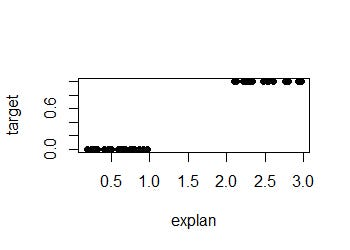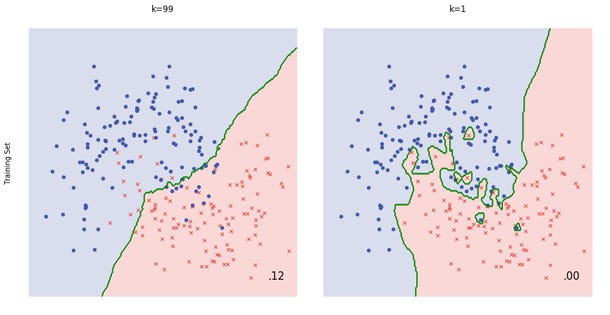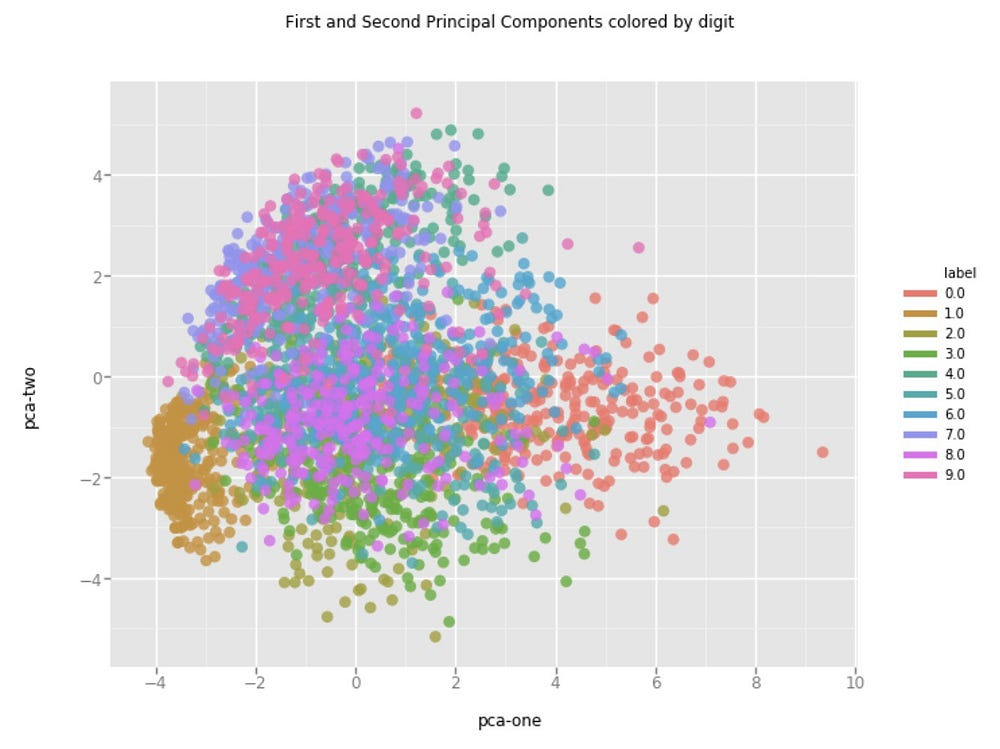# Chapter 1: K Nearest Neighbours Classifier

Introduction: K Nearest Neighbours(KNN) is one of the supervised algorithms can be used as both classifier and regressor. In this tutorial, we look at the KNN classifier. The name says Nearest Neighbours so what is that.

In the above example, there are 3 classes of class 1,2 and 3. And we need to find to which class the black point belongs to. To do that we find the nearest points to the black point. We can find the nearest points using multiple ways like Euclidean distance, Manhattan distance, the hamming distance or cosine distance. These are the widely used algorithms to calculate the distance between two points. So how many points we need to consider as neighbors? here comes K in KNN. K is a hyperparameter. Where we tune the value to maintain the balance between bias and variance. A small value of K means high variance and low bias. As K value increases the variance decreases and bias increases. (This will totally depend on how the data is distributed). Even K=1 works well without overfitting the data( consider the binary data of 0 and 1)Where K = 1 works wellLook here K = 1 overfits the data to reduce overfitting we need to increase K value

We can find nearest neighbors using 3 methods.

1. Brute force ( distance calculation)
2. KD tree
3. Locality sensitive hashing

First, we look at the Brute force method and then we follow with other methods.

First, we define the K value. How to choose the K value? The K value should be odd because we take majority voting of the nearest neighbors to define the class label. If the K value is even and we got the same majority of the classes here we can’t define which class the point belongs to( Let k=6 and we got 2 class 1, 2 class 2, 2 class 3 points as neighbors. So here we can’t decide the class label). The best practice ok K value is between 5 and 10. We can also find the best K value using parameter tuning( Checking with multiple K values and choosing the best K)

I hope you understand how KNN works. Now we discuss the Failure cases of KNN and Time complexity of the algorithm.

Failure cases:

1. When the data is random. This is the worst case of KNNJust consifer this image as KNN classifier and you can see data is completely random

2. when the data is imbalanced. If one class if 95% of the data and remaining classes belongs to 5% of the data. The predicting the class of a point always give the majority class.

Time complexity:

Let there are N points in the training set and each point is of D dimensions. There will be no time complexity for training the algorithm because we don’t do any computation in the training phase. The time complexity of the testing phase is O(N^2D) if there are N testing points. Because we need to find distance with every point in the test set with the training set and there is D dimension.

Here is the code with sci-kit learn and without sci-kit learn.

Thank you for reading

Source: Deep Learning on Medium